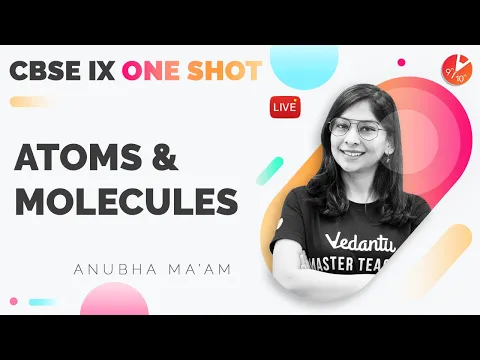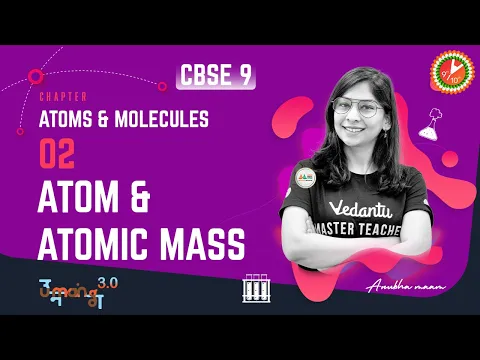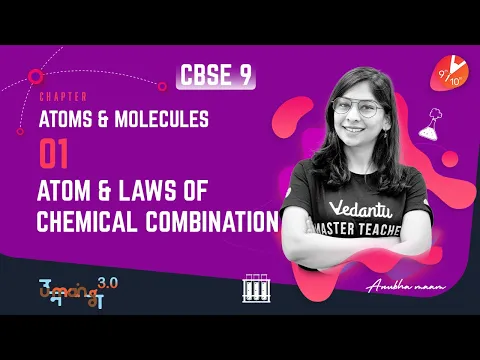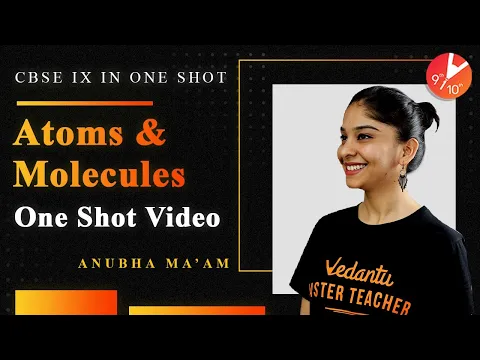Courses
Courses for Kids
Free study material
Offline Centres
More

# NCERT Solutions for Class 9 Science Chapter 3 - In HindiLast updated date: 25th Nov 2023
Total views: 424.5k
Views today: 6.24k

## NCERT Solutions for Class 9 Science Chapter 3 Atoms and Molecules in Hindi PDF Download

Download the Class 9 Science NCERT Solutions in Hindi medium and English medium as well offered by the leading e-learning platform Vedantu. If you are a student of Class 9, you have reached the right platform. The NCERT Solutions for Class 9 Science in Hindi provided by us are designed in a simple, straightforward language, which are easy to memorise. You will also be able to download the PDF file for NCERT Solutions for Class 9 Science in English and Hindi from our website at absolutely free of cost. You can also download NCERT Solutions for Class 9 Maths to help you to revise complete syllabus and score more marks in your examinations.

NCERT, which stands for The National Council of Educational Research and Training, is responsible for designing and publishing textbooks for all the classes and subjects. NCERT textbooks covered all the topics and are applicable to the Central Board of Secondary Education (CBSE) and various state boards.

We, at Vedantu, offer free NCERT Solutions in English medium and Hindi medium for all the classes as well. Created by subject matter experts, these NCERT Solutions in Hindi are very helpful to the students of all classes.

Important Topics

Topics and subtopics that are included in Class 9 Science Chapter 3 Atoms and Molecules are given as follows:

1. Atoms and Molecules

2. Laws of Chemical Combination

3. What is an Atom?

4. What is a Molecule?

5. Writing Chemical Formulae

6. Molecular Mass and Mole Concept

Watch videos on
NCERT Solutions for Class 9 Science Chapter 3 - In HindiAtoms and Molecules in One Shot(Full Chapter) | CBSE Class 9 Science Chapter 3 | Term 1 Preparation
Vedantu 9&10
SubscribeShare
3.9K likes
137.1K Views
2 years ago
Play QuizAtoms and Molecules L-2 (Atom & Atomic Mass) CBSE 9 Science Chap 3 (Chemistry)| Term 1 Exam |Vedantu
Vedantu 9&10
2.7K likes
85.7K Views
2 years agoAtoms and Molecules L-1 (𝐀𝐭𝐨𝐦 𝐚𝐧𝐝 𝐋𝐚𝐰𝐬 𝐨𝐟 𝐂𝐡𝐞𝐦𝐢𝐜𝐚𝐥 𝐂𝐨𝐦𝐛𝐢𝐧𝐚𝐭𝐢𝐨𝐧) CBSE 9 Science Chapter 3 | Vedantu
Vedantu 9&10
5.9K likes
164.7K Views
2 years agoAtoms and Molecules in One Shot | CBSE Class 9 Chemistry | Science Chapter 3 NCERT Solutions Vedantu
Vedantu 9&10
5.6K likes
170.3K Views
3 years ago
Play Quiz
See More## NCERT Solutions for Class 9 Science Chapter 3 Atoms and Molecules in Hindi

1. एक अभिक्रिया में 5.3 g सोडियम कार्बोनेट एवं 6.0 g एसिटिक अम्ल अभिकृत होते हैं। 2.2 g कार्बन डाइऑक्साइड 8.2 g सोडियम एसीटेट एवं 0.9 g जल उत्पाद के रूप में प्राप्त होते हैं। इस अभिक्रिया द्वारा दिखाइए कि यह परीक्षण द्रव्यमान संरक्षण के नियम के अनुरूप है।

सोडियम कार्बोनेट + एसिटिक अम्ल सोडियम एसीटेट + कार्बन डाइऑक्साइड + जल

उत्तरः अभिकारकों का द्रव्यमान = सोडियम कार्बोनेट का द्रव्यमान + एसिटिक अम्ल का द्रव्यमान

= 5.3 g + 6.0 g = 11.3 g

उत्पादों का द्रव्यमान = सोडियम एसीटेट + कार्बन डाइऑक्साइड + जल

= 8.2 g + 2.2 g + 0.9 g = 11.3 g

यहाँ अभिकारकों का द्रव्यमान और उत्पादों का द्रव्यमान समान है| अतः परीक्षण द्रव्यमान संरक्षण के नियम के अनुरूप है।

2. हाइड्रोजन एवं ऑक्सीजन द्रव्यमान के अनुसार 1ः8 के अनुपात में संयोग करके जल निर्मित करते हैं। 3g हाइड्रोजन गैस के साथ पूर्ण रूप से संयोग करने के लिए कितनी ऑक्सीजन गैस के द्रव्यमान की आवश्यकता होगी?

उत्तरः दिया गया है कि हाइड्रोजन और ऑक्सीजन का अनुपात = 1:8

जहाँ 1g हाइड्रोजन , 8 g ऑक्सीजन से संयोग करती है।

इसी प्रकार 3 g हाइड्रोजन होने पर वह 8 x 3 = 24 g ऑक्सीजन से संयोग करेगी।

3. डाल्टन के परमाणु सिद्धांत का कौन-सा अधिग्रहित द्रव्यमान के संरक्षण के नियम का परिणाम है ?

उत्तरः डाल्टन के परमाणु सिद्धांत का दूसरा अधिग्रहित द्रव्यमान के संरक्षण नियम का परिणाम है|जिसके अनुसार “परमाणु अविभाज्य और अत्यंत छोटे कण होते हैं जो किसी भी  रासायनिक अभिक्रिया में न तो सृजित होते हैं, न ही उनका विनाश होता है।”

4. डाल्टन के परमाणु सिद्धांत का कौन-सा अधिग्रहित निश्चित अनुपात के नियम की व्याख्या करता है?

उत्तरः डाल्टन के परमाणु सिद्धांत का 6वाँ अधिग्रहित निश्चित अनुपात के नियम की व्याख्या करता है| जिसके अनुसार “किसी भी यौगिक में परमाणुओं की सापेक्ष संख्या एवं प्रकार निश्चित होते हैं।"

5. परमाणु द्रव्यमान इकाई को परिभाषित कीजिए।

उत्तरः कार्बन-12 समस्थानिक के किसी एक परमाणु के द्रव्यमान का 1/12वें भाग का द्रव्यमान परमाणु द्रव्यमान इकाई कहलाता है।

6. एक परमाणु को आँखों द्वारा देखना क्यों संभव नहीं होता है ?

उत्तरः एक परमाणु को आँखों द्वारा देखना संभव इसलिए नहीं है क्योंकि इनका आकार बहुत  छोटा होता है। इसे सामान्यतः नैनोमीटर में मापा जाता है।

7. निम्न के सूत्र लिखिए

(i) सोडियम ऑक्साइड

उत्तरः सोडियम ऑक्साइड :

 तत्व नाम सोडियम ऑक्साइड तत्व  प्रतीक Na O आवेश +1 -2 वज्र गुणन 2 Na 1 O

सूत्र : $Na_2O$

(ii) ऐलुमिनियम क्लोराइड

उत्तरः ऐलुमिनियम क्लोराइड

 तत्व नाम एलुमिनियम क्लोराइड तत्व  प्रतीक Al Cl आवेश +3 -1 वज्र गुणन 1 Al 3 Cl

सूत्र : $AICI_3$

(iii) सोडियम सल्फाइड

उत्तरः सोडियम सल्फाइड:

 तत्व नाम सोडियम सल्फाइड तत्व  प्रतीक Na S आवेश +1 -2 वज्र गुणन 2 Al 1 S

सूत्र: $Na_2S$

(iv) मैग्नीशियम हाइड्रॉक्साइड

उत्तरः मैग्नीशियम हाइड्रॉक्साइड:

 तत्त्व नाम मैग्नीशियम हाइड्रॉक्साइड तत्त्व  प्रतीक Mg OH आवेश +2 -1 वज्र गुणन 1 Mg 2 OH

सूत्र: $Mg(OH)_2$

8. निम्नलिखित सूत्रों द्वारा प्रदर्शित यौगिकों के नाम लिखिए

(i) $Al_2(SO_4)_3$

उत्तरः एलुमिनियम सल्फेट

(ii) $CaCl_2$

उत्तरः कैल्सियम क्लोराइड

(iii) $K_2SO_4$

उत्तरः पोटेशियम सल्फेट

(iv) $KNO_3$

उत्तरः पोटेशियम नाइट्रेट

(v) $CaCO_3$

उत्तरः कैल्शियम कार्बोनेट

9. रासायनिक सूत्र का क्या तात्पर्य है?

उत्तरः जब किसी यौगिक में उपस्थित सभी तत्वों के संघटक को उनके रासायनिक प्रतीक रूप में एक साथ व्यक्त किया जाता है तो प्राप्त सूत्र को रासायनिक सूत्र तथा अणु सूत्र कहते हैं।

उदाहरण- $K_2CO_3$ एक रासायनिक सूत्र है जो पोटेशियम, कार्बन तथा ऑक्सीजन के संघटक को प्रदर्शित करता है।

10. निम्न में कितने परमाणु उपस्थित हैं?

(i) $H_2S$ अणु

उत्तरः $H_2S$  अणु में  हाइड्रोजन के 2 परमाणु तथा  सल्फर का 1 परमाणु  है।

परमाणुओं की कुल संख्या 1 + 2 = 3 है।

(ii) $PO_4^{3-}$आयन

उत्तरः $PO_4^{3-}$आयन में फास्फोरस का 1 परमाणु तथा ऑक्सीजन के 4 परमाणु हैं।

परमाणुओं की कुल संख्या 4 + 1 = 5 है।

11. निम्न यौगिकों के आण्विक द्रव्यमान की गणना कीजिए।

$H_2$, $O_2$, $Cl_2$, $CO_2$, $CH_4$, $C_2H_6$, $C_2H_4$, $NH_3$, $CH_3OH$

उत्तरः $H_2$ का आण्विक द्रव्यमान = 2 x 1 = 2 u (H परमाणु द्रव्यमान = 1 u)

$O_2$ का आण्विक द्रव्यमान = 2 x 16 = 32 u (O परमाणु द्रव्यमान = 16 u)

$Cl_2$ का आण्विक द्रव्यमान = 2 x 35.5 = 71 u (Cl परमाणु द्रव्यमान = 35.5 u)

$CO_2$ का आण्विक द्रव्यमान = 1 x 12 + 2 x 16 = 44 u (C परमाणु द्रव्यमान = 12 u)

$CH_4$ का आण्विक द्रव्यमान = 1 x 12 + 4 x 1 = 16 u

$C_2H_6$ का आण्विक द्रव्यमान = 2 x 12 + 6 x 1 = 30 u

$C_2H_4$ का आण्विक द्रव्यमान = 2 x 12 + 4 x 1 = 28 u

$NH_3$ का आण्विक द्रव्यमान = 1 x 14 + 3 x 1 = 17 u (N परमाणु द्रव्यमान =14 u)

$CH_3OH$ का आण्विक द्रव्यमान = 1 x 12 + 3 x 1 + 1 x 16 + 1 x 1 = 32 u

12. निम्न यौगिकों के सूत्र इकाई द्रव्यमान की गणना कीजिए।

ZnO, $Na_2O$, $K_2CO_3$

दिया गया है: Zn का परमाणु द्रव्यमान 65 u, Na का परमाणु द्रव्यमान = 23 u, K का परमाणु द्रव्यमान = 39 u, C का परमाणु द्रव्यमान = 12 u एवं O का परमाणु द्रव्यमान 16 u है।

उत्तरः ZnO का सूत्र इकाई द्रव्यमान = 1 x Zn का परमाणु द्रव्यमान + 1 x O का परमाणु द्रव्यमान

= 1 x 65 + 1 x 16

= 65 + 16

=  81 u

$Na_2O$ का सूत्र इकाई द्रव्यमान = 2 x सोडियम की परमाणु द्रव्यमान + 1 x ऑक्सीजन का परमाणु द्रव्यमान

= 2 x 23 + 1 x 16

= 62 u

$K_2CO_3$ का सूत्र इकाई द्रव्यमान = 2 x पोटेशियम  का परमाणु द्रव्यमान + 1 x C का परमाणु द्रव्यमान + 3 x ओक्सीजन का परमाणु द्रव्यमान

= 2 x 39 + 1 x 12 + 3 x 16

= 138

13. यदि कार्बन परमाणुओं के एक मोल का द्रव्यमान 12 g है तो कार्बन के एक परमाणु का द्रव्यमान क्या होगा ?

उत्तरः दिया गया कार्बन परमाणुओं के 1 मोल का द्रव्यमान = 12 g

तब 1 मोल कार्बन परमाणु = $6.022\times10^{23}$ कण

अतः $6.022\times10^{23}$ कार्बन परमाणुओं का द्रव्यमान = 12 g है।

1 कार्बन परमाणु का द्रव्यमान =$\frac{12}{6.022\times10^{23}}$ g

= $1.99\times10^{-23}$ g

14. किसमें अधिक परमाणु होंगे: 100 g सोडियम अथवा 100 g लोहा?  (Na का परमाणु द्रव्यमान = 23 u, Fe का परमाणु द्रव्यमान = 56 u)

उत्तरः दिया गया  सोडियम का द्रव्यमान (m) = 100 g

Na का मोलर द्रव्यमान (M) = 23 g

आवोगाद्रो संख्या No = $6.022\times 10^23$

परमाणुओं की संख्या (N) = ?

मोलों की संख्या n = m/M = 100/23 = 4.348

परमाणुओं की संख्या N = n x No

= $4.348\times 6.022\times 10^{23}$

= $2.68\times10^{24}$ परमाणु

इसी प्रकार

दिया गया लोहे का द्रव्यमान (m) = 100 g

Fe का मोलर द्रव्यमान (M) = 56 g

आवोगाद्रो संख्या No = $6.022\times10^{23}$

Fe के परमाणुओं की संख्या N = ?

n = m/M = 100/56  = 1.786

परमाणुओं की संख्या N = n x No

= $1.786\times6.022\times10^{23}$

= $1.075\times10^{24}$ परमाणु

अतः 100 g सोडियम में परमाणुओं की संख्या 100 g लोहे से अधिक है|

15. 0.24 g ऑक्सीजन एवं बोरॉन युक्त यौगिक के नमूने में विश्लेषण द्वारा यह पाया गया कि उसमें 0.096 g बोरॉन एवं 0.144 g ऑक्सीजन है। उस यौगिक के प्रतिशत संघटन का भारात्मक रूप में गणना कीजिए।

उत्तरः नमूने यौगिक का कुल भार = 0.24 g

बोरॉन का भार = 0.096 g ,ऑक्सीजन का भार = 0.144 g

बोरॉन का भारात्मक प्रतिशत = (बोरॉन का भार/यौगिक का कुल भार) x 100

= 0.096/0.24) x 100 = 40%

ऑक्सीजन का भारात्मक प्रतिशत = (0.144/0.24) x 100 = 60%

16. 3.0 g कार्बन 8.00 g ऑक्सीजन में जलकर 11.00 g कार्बन डाइऑक्साइड निर्मित करता है। जब 3.00 g कार्बन को 50.00 g ऑक्सीजन में जलाएँगे तो कितने ग्राम कार्बन डाइऑक्साइड का निर्माण होगा? आपका उत्तर रासायनिक संयोजन के किस नियम पर आधारित होगा?

उत्तरः 3.00 g कार्बन को 50.0 g ऑक्सीजन के साथ जलाने पर भी 11.00 g ही कार्बन डाइऑक्साइड प्राप्त होगी क्योंकि कार्बन और ऑक्सीजन का अनुपात (3:8) निश्चित होता हैं। इसलिये 50 g ऑक्सीजन का 8 g ऑक्सीजन ही 3.00 g कार्बन से अभिक्रिया करता है और इसमें से 50 - 8 = 42 g ऑक्सीजन शेष रह जाती है।यह स्थिर अनुपात के नियम पर आधारित  है।

17. बहुपरमाणुक आयन क्या होते हैं? उदाहरण दीजिए |

उत्तरः जब एक या एक से अधिक परमाणु आपस में मिलते हैं तब परमाणुओं का एक समूह बन जाता है, जिसे बहुपरमाणु कहते हैं और जब इस बहुपरमाणु पर कोई नेट(कुल) आवेश उपस्थित होता है, तो ये बहुपरमाणुक आयन कहलाते हैं।

जैसे- हाइड्रोक्साइड आयन जिसमे एक हाइड्रोजन और एक ऑक्सीजन परमाणु होता है और इस पर एक ऋणात्मक आयन है।

18. निम्नलिखित के रासायनिक सूत्र लिखिए।

(a) मैग्नीशियम क्लोराइड

उत्तरः मैग्नीशियम क्लोराइड

 तत्त्व नाम मैग्नीशियम क्लोराइड तत्त्व  प्रतीक Mg Cl आवेश +2 -1 वज्र गुणन 1 Mg 2 Cl

अतः सूत्र : $MgCl_2$

(b) कैल्सियम क्लोराइड

उत्तरः कैल्सियम क्लोराइड

 तत्त्व नाम कैल्सियम क्लोराइड तत्त्व  प्रतीक Ca Cl आवेश +2 -1 वज्र गुणन 1 Ca 2 Cl

अतः सूत्र : $CaCl_2$

(c) कॉपर नाइट्रेट

उत्तरः कॉपर नाइट्रेट

 तत्त्व नाम कॉपर नाइट्रेट तत्त्व  प्रतीक Cu $NO_3$ आवेश +2 -1 वज्र गुणन 1 Cu $2 NO_3$

अतः सूत्र : $Cu(NO_3)_2$

(d) ऐलुमिनियम क्लोराइड

उत्तरः ऐलुमिनियम क्लोराइड

 तत्त्व नाम ऐलुमिनियम क्लोराइड तत्त्व  प्रतीक Al Cl आवेश +3 -1 वज्र गुणन 1 Al 3 Cl

अतः सूत्र : $AlCl_3$

(e) कैल्सियम कार्बोनेट

उत्तरः कैल्सियम कार्बोनेट

 तत्त्व नाम कैल्सियम कार्बोनेट तत्त्व  प्रतीक Ca CO3 आवेश +2 -2 वज्र गुणन 2 Ca 2 CO3

अतः सूत्र : $CaCO_3$

19. निम्नलिखित यौगिकों में उपस्थित तत्वों का नाम दीजिए

(a) बुझा हुआ चूना

उत्तरः बुझा हुआ चूना = CaO

इस यौगिक में  कैल्सियम (Ca) तथा ऑक्सीजन (O) उपस्थित हैं।

(b) हाइड्रोजन ब्रोमाइड

उत्तरः हाइड्रोजन ब्रोमाइड = HBr

इस यौगिक में  हाइड्रोजन (H) तथा ब्रोमीन (Br) उपस्थित हैं।

(c) बेकिंग पाउडर (खाने वाला सोडा)

उत्तरः बेकिंग पाउडर = $NaHCO_3$

इस यौगिक में सोडियम (Na), हाइड्रोजन (H), कार्बन (C) तथा ऑक्सीजन(O) उपस्थित हैं।

(d) पोटैशियम सल्फेट

उत्तरः पोटेशियम सल्फेट= $K_2SO_4$

इस यौगिक में पोटेशियम (K), सल्फर (S) तथा ऑक्सीजन (O) उपस्थित हैं।

20. निम्नलिखित पदार्थों के मोलर द्रव्यमान का गणना कीजिए

(a) एथाइन, $C_2H_2$

उत्तरः $C_2H_2$

मोलर द्रव्यमान = 2 x 12 + 1 x 2 = 24 + 2 = 26 g

(मोलर द्रव्यमान की गणना आण्विक द्रव्यमान की तरह होती है किंतु इकाई g कर देते हैं

(b) सल्फर अणु $S_8$

उत्तरः $S_8$

मोलर द्रव्यमान = 8 x 32 = 256 g

(c) फॉस्फोरस अणु $P_4$ (फॉस्फोरस का परमाणु द्रव्यमान 31 )

उत्तरः $P_4$

मोलर द्रव्यमान = 4 x 31 = 124 g

(d) हाइड्रोक्लोरिक अम्ल, HCI

उत्तरः HCI

मोलर द्रव्यमान = 1 + 35.5 = 36.5 g

(e) नाइट्रिक अम्ल, $HNO_3$

उत्तरः $HNO_3$

मोलर द्रव्यमान 1 + 14 + 3 x 16 = 63 g

21. निम्न का द्रव्यमान क्या होगा |

(a) 1 मोल नाइट्रोजन परमाणु

उत्तरः द्रव्यमान = मोलो की संख्या x मोलर द्रव्यमान

मोलो की संख्या = 1

नाइट्रोजन परमाणु का द्रव्यमान  = 14

तब

द्रव्यमान = 1 x 14 = 14 g

(b) 4 मोल ऐलुमिनियम परमाणु (ऐलुमिनियम का परमाणु द्रव्यमान = 27)

उत्तरः मोलों कि संख्या = 4

ऐलुमिनियम का परमाणु द्रव्यमान = 27

द्रव्यमान  = मोल x परमाणु द्रव्यमान

= 4 x 27 = 108 g

(c) 10 मोल सोडियम सल्फाइट ($Na_2SO_3$)

उत्तरः $Na_2SO_3$ का मोलर द्रव्यमान  = 2 x (Na का द्रव्यमान) + 1 x S का द्रव्यमान + 3 x (O का द्रव्यमान)

= 2 x 23 + 1 x 32 + 3 x 16

= 46 + 32 + 48

= 126 g

द्रव्यमान = मोल x मोलर द्रव्यमान

= 10 x 126 = 1260 g

22. मोल में परिवर्तित किजिए

(a)12 g ऑक्सीजन गैस

उत्तरः मोलों की संख्या = दिया गया  द्रव्यमान/मोलर द्रव्यमान

1 मोल ऑक्सीजन का द्रव्यमान = मोलर द्रव्यमान = 32 g

दिया गया द्रव्यमान = 12 g

मोलो की संख्या = 12/32 = 0.375 मोल

(b) 20 g जल

उत्तरः दिया गया  द्रव्यमान = 20 g

H_{2}O मोलर द्रव्यमान= 2 x (H का द्रव्यमान) + 1 x O का द्रव्यमान

= 2 x 1 + 1 x 16

= 18 g

मोलो की संख्या = 20/18 = 1.11 मोल

(c) 22 g कार्बन डाइऑक्साइड

उत्तरः $CO_2$ का मोलर द्रव्यमान = 1 x C का द्रव्यमान + 2 x (O का द्रव्यमान)

= 1 x 12 + 2 x 16

= 44 g

दिया गया द्रव्यमान = 22 g

मोलो की संख्या = 22/44 = 0.5 मोल

23. निम्न का द्रव्यमान क्या होगा:

(a) 0.2 मोल ऑक्सीजन परमाणु

उत्तरः द्रव्यमान = मोलो की संख्या x मोलर द्रव्यमान

ऑक्सीजन परमाणु का मोलर द्रव्यमान = 16 g

मोलों की संख्या = 0.2

द्रव्यमान = 0.2 x 16 = 3.2 g

(b) 0.5 मोल जल अणु

उत्तरः द्रव्यमान = मोलो की संख्या x मोलर द्रव्यमान

जल के अणु का मोलर द्रव्यमान = 2 x (H का द्रव्यमान) + O का द्रव्यमान

= 2 x 1 + 16

= 18 g

मोलों की संख्या = 0.5

द्रव्यमान = 0.5 x 16 = 9 g

24. 16 g ठोस सल्फर में सल्फर ($S_8$) के अणुओं की संख्या का गणना कीजिए।

उत्तरः चूँकि मोलों की संख्या = दिया गया द्रव्यमान/मोलर द्रव्यमान

$S_8$ का मोलर  द्रव्यमान = 8 x (सल्फर का परमाणु द्रव्यमान)

= 8 x 32 = 256 g

तब

मोलों की संख्या  = 16/256 = 0.0625 मोल

हम जानते है कि मोलों की संख्या =अणुओं की संख्या/आवोगाद्रो संख्या

अत: अणुओं की संख्या = मोलों की संख्या x आवोगाद्रो संख्या

= $0.0625\times6.022\times10^{23}$

= $3.76\times10^{23}$

25. 0.051 g ऐलुमिनियम ऑक्साइड $Al_2O_3$ में ऐलुमिनियम आयन की संख्या का गणना कीजिए (संकेतः किसी आयन का द्रव्यमान उतना ही होता है, जितना कि उसी तत्त्व के परमाणु का द्रव्यमान होता है। ऐलुमिनियम का परमाणु द्रव्यमान 27 u है।)

उत्तरः दिया गया $Al_2O_3$ का दिया गया द्रव्यमान  = 0.051 g

1 मोल $Al_2O_3$ का द्रव्यमान = 2 x 27 + 3 x 16 = 102 g

तथा

1 मोल $Al_2O_3$ में अणुओं की संख्या = $6.022\times10^{23}$

अतः

1 मोल $Al_2O_3$ में अणुओं की संख्या = 102 g में अणुओं की संख्या = $6.022\times10^{23}$

तब

102 g में अणुओं की संख्या = $6.022\times10^{23}$

अतः 1 g में अणुओं की संख्या = $\frac{6.022\times10^{23}}{102}$

0.051 g $Al_2O_3$  में अणुओं की संख्या = $0.051\times6.022\times10^{23}$

= $3.011\times10^{20}$

चुँकि ऐलुमिनियम ऑक्साइड के एक अणु में Al के 2 आयन होते है|

अतः कुल ऐलुमिनियम आयन की संख्या = $2\times3.011\times10^{20}$  = $6.022\times10^{20}$

## NCERT Solutions for Class 9 Science Chapter 3 Atoms and Molecules in Hindi

Chapter-wise NCERT Solutions are provided everywhere on the internet with an aim to help the students to gain a comprehensive understanding. Class 9 Science Chapter 3 solution Hindi medium is created by our in-house experts keeping the understanding ability of all types of candidates in mind. NCERT textbooks and solutions are built to give a strong foundation to every concept. These NCERT Solutions for Class 9 Science Chapter 3 in Hindi ensure a smooth understanding of all the concepts including the advanced concepts covered in the textbook.

NCERT Solutions in Hindi medium have been created keeping those students in mind who are studying in a Hindi medium school. These NCERT Solutions for Class 9 Science Atoms and Molecules in Hindi medium pdf download have innumerable benefits as these are created in simple and easy-to-understand language. The best feature of these solutions is a free download option. Students of Class 9 can download these solutions at any time as per their convenience for self-study purpose.

These solutions are nothing but a compilation of all the answers to the questions of the textbook exercises. The answers/solutions are given in a stepwise format and very well researched by the subject matter experts who have relevant experience in this field. Relevant diagrams, graphs, illustrations are provided along with the answers wherever required. In nutshell, NCERT Solutions for Class 9 Science in Hindi come really handy in exam preparation and quick revision as well prior to the final examinations.

### NCERT Solutions for Class 9 Science in Hindi

Important Points

• The relative number and types of atoms in a particular compound are constant, according to Dalton's atomic theory, which is based on the rule of conservation of mass. In a chemical reaction, atoms cannot be generated or destroyed.

• A compound's relative quantity and types of atoms remain constant.

• One atomic mass unit is one-twelfth (1/12th) of the mass of one carbon-12 atom. All of the elements' relative atomic masses have been discovered in relation to a carbon-12 atom.

• Atoms are so minuscule that they can't be seen with the human eye. Nanometres are the units of measurement. 1 meter equals 109 nanometers.

• The chemical formula of a compound is a symbolic representation of its composition, for example, sodium chloride has the chemical formula NaCl.

## FAQs on NCERT Solutions for Class 9 Science Chapter 3 - In Hindi

1. Define the atomic mass unit.

We can define it as 1/12 of the mass of a carbon-12 atom. One AMU is the average of the proton rest mass and the neutron rest mass, to put it in the simplest words. It is a mass unit used in physics and chemistry. Atomic and molecular masses are expressed by this unit of mass. About 1.67375 x 10-27 kgs or 1.67375 x 10-24 gms are involved in this measurement.

2. What is meant by the term chemical formula?

There are several ways to convey information about the chemical proportions of the atoms in a given compound, such as using chemical element symbols and numbers, as well as occasionally additional symbols such as parentheses etc. They reveal the components that make up a compound's molecules as well as the ratios in which these elements mix to produce those molecules. It is nothing but a chemical symbolization.

3. What basically are atoms and molecules?

"Atom" is the scientific term for the fundamental unit of matter, which is an electron cloud encircling the dense nucleus. Chemical covalent bonds hold together two or more atoms to form molecules. It is possible to form molecules from the same atoms or from atoms that are distinct from one another. They are termed molecules of elements if they are all composed of the same type of atoms.

4. Who gave the name atom Class 9?

Democritus, a great philosopher, coined the term. Further on, he went on to explain that matter is made up of tiny particles called atoms that can't be separated from each other. As a result, Democritus invented the word atomos, which signifies indivisible. This is how the word Atom came into being in modern-day science. These atoms form a crucial domain of study in science.

5. What is inside an atom?

Protons, electrons, and neutrons are the three fundamental particles that make up an atom. Positively charged protons and neutrons are found in the atom's nucleus (no charge). Electron shells are the outermost portions of the atom and contain electrons (-ve charge). This is the basic composition of any atom. Atoms consist of a small nucleus surrounded by electrons that are constantly in motion.